The properties of vectors, attraction and rejection force, show that the substance is electricity.
The organizing and operation of vectors in oscillators is therefore suggestive.
The attraction force among vectors is similar to that of electric current, and the rejection force, like tension.
The vector being component of space can be interpreted as depression/pressure as well, or absorption / emission.
In the drawings above, current oscillation induces alternatively the variation of current in the two rings.
Tension oscillation induces alternatively the variation of voltage in the two rings. In case of travelling oscillator, the variation of tension propagates the rings ne through the other, alternatively and orthogonally.
Actually, oscillations are mixed, energy being the product of the variation of attraction and rejection forces (UI).
The reasoning is applied as well to the gravitational phenomenon, considering it similar to product of attraction and rejection forces.
The gravitational phenomenon is different, the UI product being focused towards central point !!!
Therefore, there is an imbalance between the periphery and the central area, where the acceleration is changed in perpetual pressure !!!
How is that, pressure?

"Compressor", the pump, is gravitational acceleration, polarized space, attraction and rejection force of vectors.
"Receiver" is sun with all the system, the row of spheres of vectors which propagate pressure strength to the center.
In the drawing below, the graphic of gravitational acceleration presents a logical limit.
Inside the sun (star), gravitation gathers the entire energy of the system.
Here, in the focal, gravity produces the maximum pressure, density and temperature limits (with Eruptions! Jets!).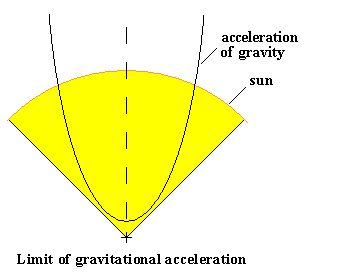Gravity is electric induction
Radiations from far away galaxies show spectral traveling.
Conclusion, galaxies get further away (the universe is dilating!). There is, however, absurd reciprocity, for the space to get closer and not galaxies to get further away (the universe is rotating!)
Spectral traveling has as well light which goes away or comes from a star, cause attributed to gravity. There is here as well absurd reciprocity, that space gets closer to the star.
Therefore, the cause of spectral traveling can be "space" propagation to the rotation center!
Space consists of existence vectors - FLUEN
Vectors have attraction forces - CURRENT
And rejection forces - TENSION
Such forces propagate their orientation with "c" speed and produce oscillations -electromagnetic waves. Rotation movement generates electric induction, orienting the current (attraction forces) in a curve shape, which propagate in convergence to the focal of the curve.
This is gravity. A simple phenomenon, but with many and complex consequences.
The phenomenon is similar as well in the propagation of tension (rejection forces)
Tension methodized on curve surface divergently propagate polarity orientation which is opposed to the curve focal.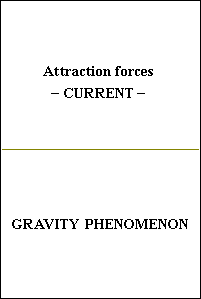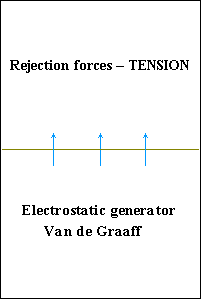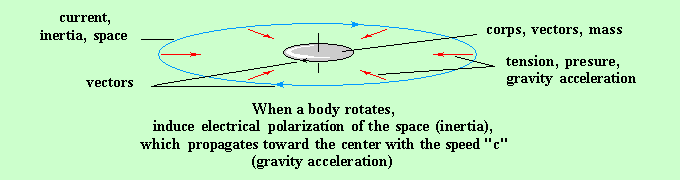The mass is the size relationship between the vectors of the body and the vectors of the space.
The inertia are the interactions between the body vectors and vectors of space (between mass and space)
Gravity comes from inertie!

>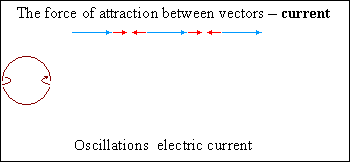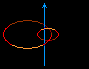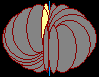Oscillations Mixed - current and tension